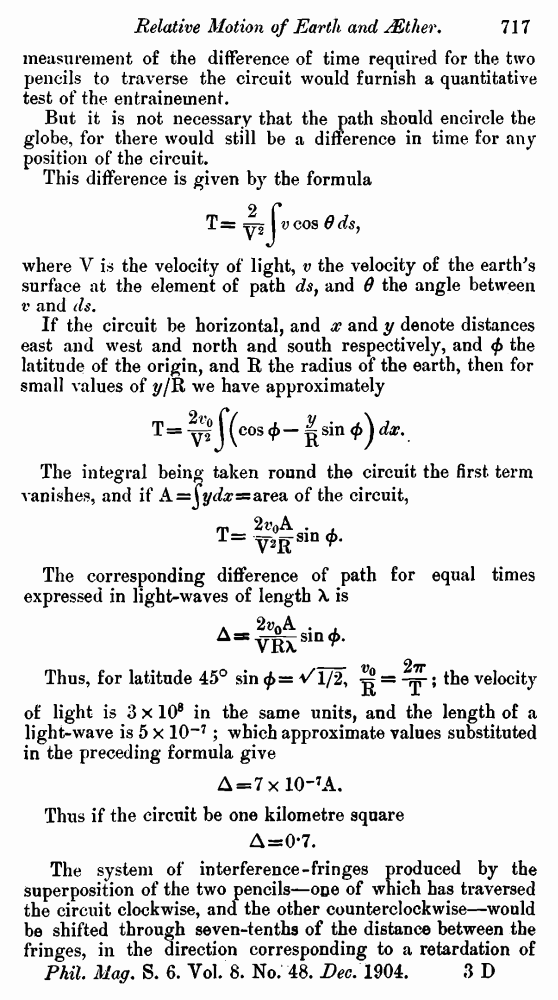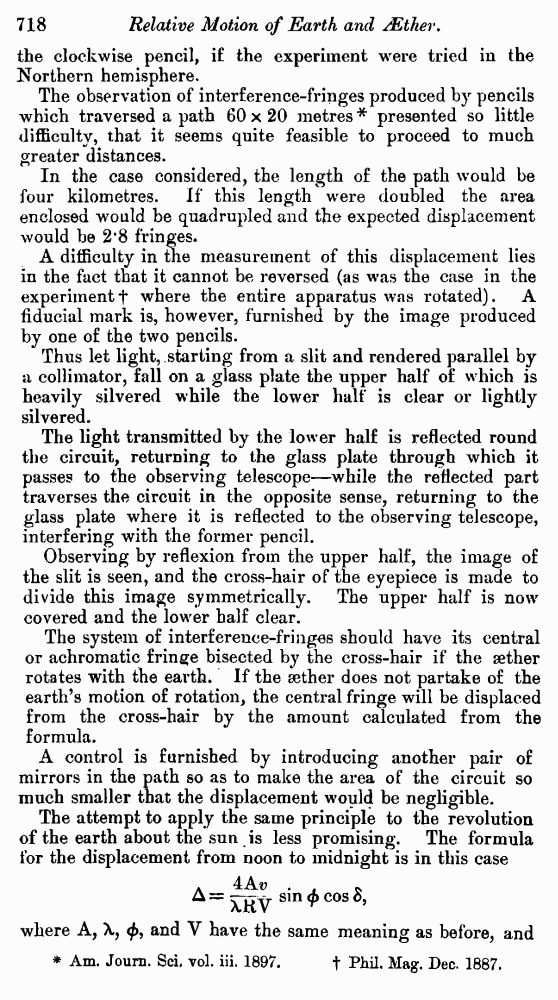﻿ A. A. Michelson. Relative Motion of Earth and AEther // Phil. Mag. S. 6. Vol. 8. No. 48. Dec. 1904.

# A. A. Michelson. Relative Motion of Earth and AEther // Phil. Mag. S. 6. Vol. 8. No. 48. Dec. 1904.

В начало   Другие форматы   <<<     Страница 717   >>>716  717 718  719 measurement of the difference of time required for the two pencils to traverse the circuit would furnish a quantitative test of the entrainement. But it is not necessary that the path should encircle the globe, for there would still be a difference in time for any position of the circuit. This difference is given by the formula where V is the velocity of light, v the velocity of the earth's surface at the element of path ds, and θ the angle between v and ds. If the circuit be horizontal, and x and y denote distances east and west and north and south respectively, and φ the latitude of the origin, and R the radius of the earth, then for small values of y/R we have approximately The integral being taken round the circuit the first term vanishes, and if A=∫ ydx = area of the circuit, The corresponding difference of path for equal times expressed in light-waves of length λ is Thus, for latitude 45°the velocity of light is 3 × 108 in the same units, and the length of a light-wave is 5 × 10–7; which approximate values substituted in the preceding formula give Δ = 7 × 10–7A. Thus if the circuit be one kilometre square Δ=0.7. The system of interference-fringes produced by the Superposition of the two pencils—one of which has traversed the circuit clockwise, and the other counterclockwise—would be shifted through seven-tenths of the distance between the fringes, in the direction corresponding to a retardation of Phil. Mag. S. 6. Vol. 8. No. 48. Dec. 1904. 3 D the clockwise pencil, if the experiment were tried in the Northern hemisphere. The observation of interference-fringes produced by pencils which traversed a path 60 × 20 metres* presented so little difficulty, that it seems quite feasible to proceed to much greater distances. In the case considered, the length of the path would be four kilometres. If this length were doubled the area enclosed would be quadrupled and the expected displacement would be 2.8 fringes. A difficulty in the measurement of this displacement lies in the fact that it cannot be reversed (as was the case in the experiment † where the entire apparatus was rotated). A fiducial mark is, however, furnished by the image produced by one of the two pencils. Thus let light, starting from a slit and rendered parallel by a collimator, fall on a glass plate the upper half of which is heavily silvered while the lower half is clear or lightly silvered. The light transmitted by the lower half is reflected round the circuit, returning to the glass plate through which it passes to the observing telescope—while the reflected part traverses the circuit in the opposite sense, returning to the glass plate where it is reflected to the observing telescope, interfering with the former pencil. Observing by reflexion from the upper half, the image of the slit is seen, and the cross-hair of the eyepiece is made to divide this image symmetrically. The upper half is now covered and the lower half clear. The system of interference-fringes should have its central or achromatic fringe bisected by the cross-hair if the aether rotates with the earth. If the aether does not partake of the earth’s motion of rotation, the central fringe will be displaced from the cross-hair by the amount calculated from the formula. A control is furnished by introducing another pair of mirrors in the path so as to make the area of the circuit so much smaller that the displacement would be negligible. The attempt to apply the same principle to the revolution of the earth about the sun is less promising. The formula for the displacement from noon to midnight is in this case where A, λ, φ, and V have the same meaning as before, and * Am. Journ. Sci. vol. iii. 1897. † Phil. Mag. Dec. 1887.